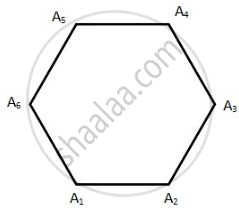Advertisement Remove all ads

# Three of the six vertices of a regular hexagon are chosen at random. What is the probability that the triangle with these vertices is equilateral. - Mathematics

Three of the six vertices of a regular hexagon are chosen at random. What is the probability that the triangle with these vertices is equilateral.

Advertisement Remove all ads

#### Solution

We choose three vertices out of 6 in 6C3 = 20 ways.
∴ Total number of elementary events = n(S) = 20
Number of ways of choosing an equilateral triangle = 2i.e. A1A3A5 or A2A4A6
∴ Total number of favourable events = n(E) = 2
Hence, required probability = $\frac{2}{20} = \frac{1}{10}$

Is there an error in this question or solution?
Advertisement Remove all ads

#### APPEARS IN

RD Sharma Class 11 Mathematics Textbook
Chapter 33 Probability
Q 8 | Page 71
Advertisement Remove all ads

#### Video TutorialsVIEW ALL 

Advertisement Remove all ads
Share
Notifications

View all notifications

Forgot password?